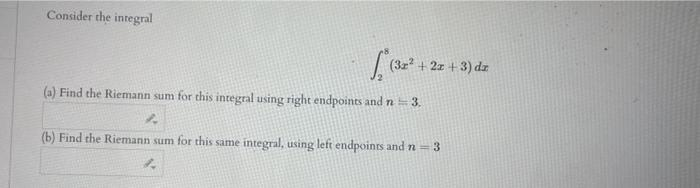# (Solved): Consider the inregral $\int_{2}^{8}\left(3 x^{2}+2 x+3\right) d x$ (a) Find the Riemann sum for ...Consider the inregral $\int_{2}^{8}\left(3 x^{2}+2 x+3\right) d x$ (a) Find the Riemann sum for this integral using right endpoints and $$n=3$$. (b) Find the Riemann sum for this same integral, using left endpoinss and $$n=3$$

We have an Answer from Expert